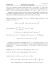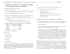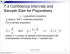# Chapter 8: STATISTICAL INTERVALS FOR A SINGLE SAMPLE Part 3:

## Transcription

Chapter 8: STATISTICAL INTERVALS FOR A SINGLE SAMPLE Part 3:
```Chapter 8: STATISTICAL INTERVALS
FOR A SINGLE SAMPLE
Part 3:
• Summary of CI for µ
• Confidence Interval for a Population
Proportion p
Section 8-4
Summary for creating a 100(1-α)% CI for µ:
• When σ 2 is known and parent population is
normal, use a z-value (this works for any n).
x¯ ± zα/2 · √σn
• When σ 2 is unknown and n is REALLY large,
use a z-value and replace σ 2 with the observed sample variance s2 (this works for any
parent population distribution).
x¯ ± zα/2 · √sn
1
• When σ 2 is unknown and n is relatively small,
and POPULATION IS ‘NEARLY’ NORMAL,
use a t-value and the sample variance.
x¯ ± tα/2,n−1 · √sn
• Before you make a CI, it is a random interval... it depends on the sample chosen, but
¯ will ALWAYS be at the center of the CI.
X
Example of 16 CI’s for µ each based on a
different sample:
2
• Example: Fuel rods in a reactor (problem
8-41 in book)
An article in Nuclear Engineering International gives the following measurements on
the percentage of enrichment of 12 fuel rods
in a reactor in Norway:
2.94
2.90
3.00
2.75
2.90
2.95
2.75
2.82
3.00
2.81
2.95
3.05
Calculate a 95% CI for the mean percentage
of enrichment. Provide a normal probability
plot to verify the assumption of normality.
ANS: This is a small sample with unknown
σ 2, so we will use the procedure with the tvalue.
x¯ ± tα/2,n−1 · √sn
3
x¯=2.9017
s2=0.0098
s = 0.0993
tα/2,n−1 = t0.025,12−1 = t0.025,11 = 2.201
95% CI for µ:
√
2.9017 ± 2.201 · (0.0993/ 12)
2.9017 ± 0.0631
[2.8386, 2.9648]
4
Checking normality with a normal probability plot:
normal probability plot
1.5
●
1.0
●
0.5
●
●
0.0
●
●
●
−0.5
Theoretical Quantiles
●
●
●
−1.5
−1.0
●
●
2.75
2.80
2.85
2.90
2.95
3.00
3.05
Sample Quantiles
Looks pretty good. The points fall randomly
around the diagonal line. So, we can believe
that the data was generated from a normal
distribution (i.e. the parent population was
at least ‘approximately’ normal).
5
Population Proportion Parameter p
• Moving on to another potential parameter of
interest...
A population proportion is denoted p.
A sample proportion is denoted pˆ.
• A population proportion is based on a yes/no
or 0/1 type variable.
– What proportion of the population favor
Mitt Romney?
yes/no
– What proportion of a manufactured good
is defective?
defective/not defective
6
– What proportion of the U.S. is republican?
republican/not republican
– What proportion of students entering college successfully complete a degree?
succeed/fail
• To estimate a population proportion, we will
use a sample proportion.
7
Large-Sample Confidence Interval for
a Population Proportion p
Section 8-4
• The construction of the CI for p relies on the
fact that we took a large sample (large n).
• I will introduce this concept using something
we are familiar with, the binomial...
– We will let the category of interest be called
the ‘success’ category (arbitrary).
– Let Xi = 1 if observation i falls into the
‘success’ category.
– Let Xi = 0 if observation i falls into the
other or ‘fail’ category.
– Xi is called an indicator variable.
8
– Thus,
Pn
i=1 Xi = a count of all individuals in
the ‘success’ category.
– The sample proportion of individuals falling
into the ‘success’ category is
Pn
Xi
i=1
ˆ
P =
n
# in sample who are in ‘success’ category
=
n
– Pˆ (upper case) is a random variable and
is the point estimator for p.
– pˆ (lower case) is a realized point estimate
from a observed sample.
9
• Note that p and n are actually the parameters for a binomial distribution (i.e. probability of a success and number of trials).
– There are n trials (i.e. n independent draws
of individuals to form the sample)
– The probability of getting a ‘success’ remains constant as p (assuming we have a
large population and n is not too large)
– Let Y =total number of successes.
– So Y ∼ Binomial(n, p)
– E(Y ) = np
and
V (Y ) = np(1 − p)
– In our notation, Y =
Y =
Pn
Pn
i=1 Xi, so
i=1 Xi ∼ Binomial(n, p)
10
E(
Pn
i=1 Xi) = np
and
Pn
V ( i=1 Xi) = np(1 − p)
• Thus, if n is large, we have
Pn
Pn
( i=1 Xi)
−p
( i=1 Xi) − np
n
Z= p
= q
p(1−p)
np(1 − p)
n
Pˆ − p
=q
p(1−p)
n
where Z is approximately standard normal.
(NOTE: This is a normal approximation to
the binomial. And for the approximation to
be reasonable, we should have np ≥ 5 and
n(1 − p) ≥ 5.)
11
• Normal approximation for a sample
proportion Pˆ
If n is large, the distribution of
Pˆ − p
Z=q
p(1−p)
n
is approximately standard normal.
Or similarly,
p(1−p)
ˆ
P ∼ N (p, n )
This means the sampling distribution for Pˆ
is normal...
See applet at:
http://www.rossmanchance.com/applets/Reeses/ReesesPieces.html
12
Thus, we can can use a z-value to form a
100(1-α)% CI for p:
Pˆ − p
≤ zα/2) = 1 − α
P (−zα/2 ≤ q
p(1−p)
n
rearranging...
r
P
Pˆ − zα/2
p(1 − p)
≤ p ≤ Pˆ + zα/2
n
r
p(1 − p)
n
!
=1−α
and we have the lower and upper bounds...
q
p(1−p)
Lower bound: Pˆ − zα/2
q n
p(1−p)
Upper bound: Pˆ + zα/2
n
BUT WE DON’T KNOW p, SO WE CAN’T
GET ACTUAL VALUES FOR THE BOUNDS!
Solution ⇒ replace p with Pˆ in the formulas.
13
• Approximate 100(1-α)% CI
for a population proportion p
If pˆ is the proportion of observations in a
random sample of size n that belongs to a
class of interest, an approximate 100(1-α)%
CI on the proportion of p of the population
that belongs to this class is
r
pˆ(1 − pˆ)
pˆ ± zα/2
n
where zα/2 is the upper α/2 percentage point
of the standard normal distribution.
• Things you need for the appropriate behavior
of Pˆ :
– Population is large, and you don’t take too
many individuals for your sample. Maybe
no more than 10% of the total population.
14
– The sample is a simple random sample.
– np ≥ 5 and n(1 − p) ≥ 5.
This statement means that if you have a
really rare event, you’re going to need a
very large sample... just logical though.
• Example: Interpolation methods are used
to estimate heights above sea level for locations where direct measurement are unavailable.
After verifying the estimates, it was found
that the interpolation method made “large”
errors at 26 of 74 random sample test locations.
Find a 90% CI for the overall proportion
of locations at which this method will make
“large” errors.
15
ANS:
16
• Choice of sample size for
estimating p
For a specified error E = |p − Pˆ | in your
estimate, the previously stated behavior of
Pˆ suggests you should choose a sample size
as:
zα/2 2
n=
· p(1 − p)
E
But since we don’t know p (that’s what we’re
trying to estimate!), we can’t compute a sample size from this formula... unless we
estimate p first.
Here, we choose to err on the conservative
side. It turns out the largest variance for Pˆ
p(1−p)
ˆ
[where V (P ) = n ] occurs when p = 0.5
no matter what the n was:
17
0.004
0.000
0.002
p(1−p)/n
0.006
0.008
Plot of variance of Pˆ vs. p
0.0
0.2
0.4
0.6
0.8
1.0
p
So, if we don’t know p, we’ll plug-in p =
0.5 to make sure we don’t under-estimate the
variance of our estimate (using ‘worst case
scenario’ idea).
18
• Thus, the working sample size formula for
estimating p with a 100(1 − α)% CI and an
error of E is:
zα/2 2
0.25
n=
E
We use this method because, before we collect data, we don’t have any information on
p, so there’s no observed pˆ to plug-in.
In contrast, when doing a CI for p, we DO
have an observed estimate pˆ for p, so in that
case, so use our plug-in estimate.
19
• Example: In the interpolation example, how
large of a sample would you need if you wanted
to be at least 95% confident that the error
in your estimate (i.e. |p−pˆ|) is less than 0.08?
ANS:
20
• Example: Gallup Poll
Between February 9-11, 2007, adults were
randomly sampled (by phone) and asked:
“Would you favor or oppose Congress taking action to set a time-table for withdrawing all U.S. troops from Iraq by the end of
next year.”
Let p = the proportion of the population
wanting a withdrawal time-table.
How large is their error if they sample 1000
people and form a a 95% CI?
21
ANS:
See USA Today, February 12, 2007.
http://www.usatoday.com/news/polls/tables/live/2007-02-12-poll.htm
22
```

### STANDARD NORMAL DISTRIBUTION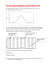### Sample size Student Learning Centre Semester 2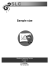### DETERMINING SAMPLE SIZE FOR RESEARCH ACTIVITIES ROBERT V. KREJCIE### measure attention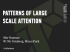### Millennium Development Goals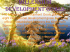### Proportion Power Point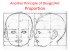### Chapter 8-Principles of Design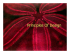### health - Dental Health Services Victoria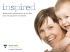### 12 Solving Percents Using Proportions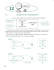### 1-4 Solving Proportions - Harmony Public Schools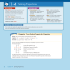### MATH 382 Chebyshev`s Inequality